# SAT Math Multiple Choice Question 890: Answer and Explanation

### Test Information

Question: 890

5.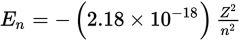Electrons follow paths, called orbits, around the nucleus of an atom. According tothe Bohr model, the energy in joules of an atom's nth orbit containing a single electron is given by the formula shown above, where Z is the atomic number of the atom and n is the orbit number. Which of the following equations gives the atomic number ofan atom given the energy of its nth orbit (assuming there is a single electron in that orbit)?

• A.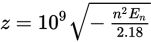• B.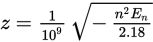• C.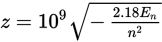• D.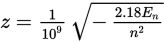Explanation:

A

Difficulty: Hard

Category: Passport to Advanced Math / Exponents

Strategic Advice: A question like this requires patience and concentration. As with any equation, use inverse operations to solve for (isolate) the desired variable.

Getting to the Answer: To solve the equation for Z, start by multiplying both sides of the equation by the only expression that's in the denominator on the right-hand side (n2). Then, divide by everything that Z is being multiplied by (-2.18 × 10-18). To remove the negative exponent, you can move 10-18 to the numerator (but not 2.18 because it is not being raised to a negative power):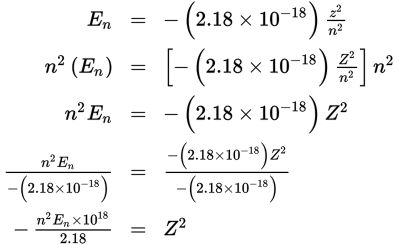You're asked for Z (not Z 2), so take the square root of both sides of the equation to find that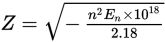. This is not one of the answer choices, but you can take the square root of 1018 by writing it as (109)2, which results in the equation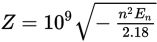. This means (A) is correct.

Note: You may recall that you can't take the square root of a negative number, but electron energy is given as a negative number, which means you're multiplying a negative number by another negative number under the radical, resulting in a positive quantity.